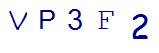# Decoding 1st Grade Math Word ProblemsLast Updated on August 31, 2021 by Thinkster

In first grade, children are introduced to math word problems. These problems are simple, but they may end up becoming a challenge for your child. Here are the steps to decode 1st grade math word problems, so they are easy to handle for your first grader.

## 1. Draw a Picture

One of the reasons 1st grade math word problems are so challenging is the fact that these students are still learning to decode the written word. When they can’t figure out what is being asked, they can’t solve the problem. So, teach your child to draw a picture. The good news is that the numbers in first grade math are still relatively small, so you can easily draw a picture to illustrate most problems.

## 2. Identify the Question

When solving 1st grade math word problems, you must identify the question. This is rarely hard to do with early word problems. Find the question mark, then see what is being asked.

## 3. Find the Numbers

Next, the student needs to find the numbers. When these are written in numerical form, it’s fairly easy. When they are written as words, it requires a bit more decoding. Help your child learn to identify and write down the numbers by the word in order to solve the problem.

## 4. Look for Clue Words

Math word problems always include clue words. These tell the student what to do to solve the problem. Your child needs to know the clue words commonly found in 1st grade math word problems, identify them and determine what to do with them.

Because most 1st grade math word problems are subtraction or addition problems, these are the words your child needs to know:

• Subtraction: Difference, how many more, how much greater, subtracted from, minus

## 5. Write the Equation

Teach your child to use the clue and number words to write an equation. Writing down what they are supposed to do will help students make the right choice about the operation to use. After writing the equation, the child should solve it.

## 6. Ask, Does It Make Sense?

Finally, before moving on from the word problem, the child should consider whether or not the answer makes sense. An answer that is logical and in line with what the question is asking is likely the right answer. An answer that is way too large or way too small may indicate that the wrong operation was used.

If you find that 1st grade math word problems are throwing your child for a loop, now is the time to act. First graders need a solid foundation in math if they are going to succeed as they progress through math classes. Use a program, like Thinkster Math, that is engaging and easy to follow to reinforce important math concepts, including story problems, at home. By plugging into tablet-based instruction, you can enjoy the help of certified instructors helping your child master and excel in 1st grade math word problems.

SummaryArticle Name
Decoding 1st Grade Math Word Problems
Description
Here are the steps to decode 1st grade math word problems, so they are easy to handle for your new first grader.
Author
Publisher Name
Thinkster Math
Publisher Logo

## Subscribe to Thinkster Blog## Recommended Articles### Unlocking the Code: Teaching Mathematical Symbols and Their Meaning

Knowledge of mathematical symbols is a foundational skill that empowers students to engage with and excel i...